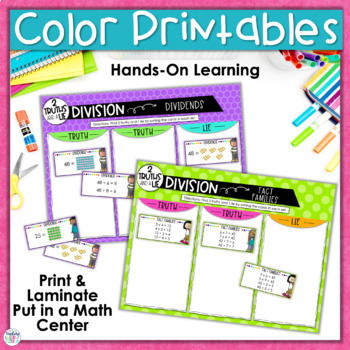# Division Error Analysis Activity | 2 Truths and a Lie2nd - 4th
Subjects
Standards
Resource Type
Formats Included
• Zip
•Google Apps™
Pages
20 pagesThe Teacher-Author indicated this resource includes assets from Google Workspace (e.g. docs, slides, etc.).

### Description

Your students will love this fun and engaging hands-on division activity that helps them practice division strategies such as equal groups, arrays, and fact families through error analysis! This QUICK PRINT AND GO or DIGITAL activity also gives students important practice with math vocabulary.

How to Use this Division Activity:

Students are given 3 division representations (example: arrays, equal groups, fact families) and must determine which 2 are true and which one is a lie.

⭐ There are 5 different activities in this resource that help students practice with arrays, equal groups, fact familes, and using math vocabulary (dividends, divisors & quotients).

⭐ This 2 Truths and a Lie Division Error Analysis activity has so much flexibility in a printer friendly black and white version or color printables, and a digital version in Google™ Slides. Use it whichever way is best for YOU and your STUDENTS:

★ Cut and paste math centers activity

★ Print and laminate the pieces and use multiple times

★ Assign the digital version in a computer center.

→→→ PLEASE SEE PREVIEW FOR A CLOSER LOOK!

───────────────────────────

What's Included in this Resource:

Two Truths and a Lie Organizer Sheet, Division Representation Cards, and Answer Key for:

• Arrays
• Equal Groups
• Fact Families
• Dividends
• Divisors & Quotients

───────────────────────────

2 Truths and a Lie Division Activities can easily be used in these settings:

• Whole Group Instruction
• Small Group Instruction
• Centers
• Formative Assessment
• Partner Work
• Bell Work

───────────────────────────
Common Core Math Standards Addressed in this Resource:
3.OA.A.1 - Interpret products of whole numbers, e.g., interpret 5 × 7 as the total number of objects in 5 groups of 7 objects each.
3.OA.A2 - Interpret whole number quotients of whole numbers, e.g., interpret 56 ÷ 8 as the number of objects in each share when 56 objects are partitioned equally into 8 shares, or as a number of shares when 56 objects are partitioned into equal shares of 8 objects each.
3.OA.B5 - Apply properties of operations as strategies to multiply and divide.

───────────────────────────
If you and your students love this fun and engaging error analysis activity for division, you will love these too!

Need more engaging and rigorous division resources?

"Don't forget to leave feedback -you will receive TPT credits that you can use toward future purchases!

Thank you for visiting Teaching in the Heart of Florida!

Total Pages
20 pages
Included
Teaching Duration
Other
Report this Resource to TpT
Reported resources will be reviewed by our team. Report this resource to let us know if this resource violates TpT’s content guidelines.

### Standards

to see state-specific standards (only available in the US).
Interpret products of whole numbers, e.g., interpret 5 × 7 as the total number of objects in 5 groups of 7 objects each. For example, describe a context in which a total number of objects can be expressed as 5 × 7.
Interpret whole-number quotients of whole numbers, e.g., interpret 56 ÷ 8 as the number of objects in each share when 56 objects are partitioned equally into 8 shares, or as a number of shares when 56 objects are partitioned into equal shares of 8 objects each. For example, describe a context in which a number of shares or a number of groups can be expressed as 56 ÷ 8.
Apply properties of operations as strategies to multiply and divide. Examples: If 6 × 4 = 24 is known, then 4 × 6 = 24 is also known. (Commutative property of multiplication.) 3 × 5 × 2 can be found by 3 × 5 = 15, then 15 × 2 = 30, or by 5 × 2 = 10, then 3 × 10 = 30. (Associative property of multiplication.) Knowing that 8 × 5 = 40 and 8 × 2 = 16, one can find 8 × 7 as 8 × (5 + 2) = (8 × 5) + (8 × 2) = 40 + 16 = 56. (Distributive property.)